Courses

# Test: Geometry- 1

## 15 Questions MCQ Test UPSC Prelims Paper 2 CSAT - Quant, Verbal & Decision Making | Test: Geometry- 1

Description
This mock test of Test: Geometry- 1 for UPSC helps you for every UPSC entrance exam. This contains 15 Multiple Choice Questions for UPSC Test: Geometry- 1 (mcq) to study with solutions a complete question bank. The solved questions answers in this Test: Geometry- 1 quiz give you a good mix of easy questions and tough questions. UPSC students definitely take this Test: Geometry- 1 exercise for a better result in the exam. You can find other Test: Geometry- 1 extra questions, long questions & short questions for UPSC on EduRev as well by searching above.
QUESTION: 1

### A vertical stick 20 m long casts a shadow 10 m long on the ground. At the same time, a tower casts the shadow 50 m long on the ground. Find the height of the tower.

Solution:

When the length of stick = 20 m, then length of shadow = 10 m i.e. in this case length = 2 X shadow.

With the same angle of inclination of the sun, the length of tower that casts a shadow of 50 m fi 2 X 50m = 100m i.e. height of tower = 100 m

QUESTION: 2

### The area of similar triangles, ABC and DEF are144cm2 and 81 cm2 respectively. If the longest side of larger △ABC be 36 cm, then the longest side of smaller △DEF is

Solution: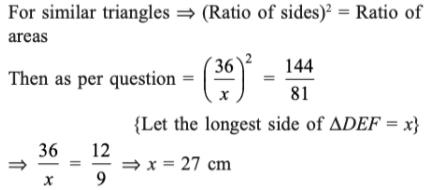QUESTION: 3

### Find the value of x in the figure, if it is given that AC and BD are diameters of the circle.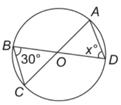Solution:

The triangle BOC is an isosceles triangle with sides OB and OC both being equal as they are the radii of the circle. Hence, the angle OBC = angle OCB = 30°.

Hence, the third angle of the triangle BOC viz: Angle BOC would be equal to 120°. Also, BOC = AOD = 120°. Hence, in the isosceles triangle DOA, Angle ODA = Angle DAO = x = 30°.

QUESTION: 4

Find the value of x in the given figure.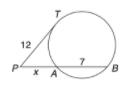Solution:

By the rule of tangents, we get
122 = (x + 7)x -> 144 = x2 + 7x
=> x2 + 7x – 144 = 0 -> x2 +16x – 9x –144 = 0
=> x(x + 16) – 9(x + 16)
=> x = 9 or –16
–16 can’t be the length, hence this value is discarded,
thus, x = 9

QUESTION: 5

Find the value of x in the given figure.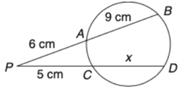Solution:

By the rule of chords, cutting externally, we get

=> (9 + 6)6 = (5 + x)5

=> 90 = 25 + 5x

=> 5x = 65
=> x = 13cm

QUESTION: 6

In the given figure ㄥPAB = 25deg. AB is the diameter. Find ㄥTPA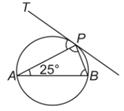Solution:

ㄥAPB = 90° (angle in a semicircle = 90°)

ㄥPBA =180 – (90 + 25) = 65°

ㄥTPA = ㄥPBA (the angle that a chord makes with the tangent, is subtended by the chord on the circumference in the alternate segment) = 65°
Note: This is also called the  Alternate Segment Theorem.

QUESTION: 7

In the figure, AB is parallel to CD and RD || SL || TM || AN, and BR : RS : ST : TA = 3 : 5 : 2 : 7. If it is known that CN = 1.333 BR. Find the ratio of BF : FG : GH : HI : IC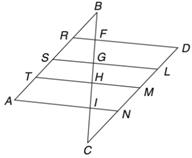Solution:

Since the lines AB and CD are parallel to each other, and the lines RD and AN are parallel, it means that the triangles RBF and NCI are similar to each other. Since the ratio of CN : BR = 1.333, if we take BR as 3, we will get CN as 4.

This means that the ratio of BF : CI would also be 3 : 4. Also, the ratio of BR : RS : ST : TA = BF : FG : GH : HI = 3 : 5 : 2 : 7 (given). Hence, the correct answer is 3 : 5 : 2 : 7 : 4.

QUESTION: 8

In the following figure, it is given that O is the centre of the circle and ㄥAOC = 140°. Find ㄥABC.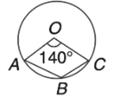Solution: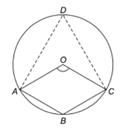∠AOC=140° in the figure

we have to find the value of x

∠AOC of minor sector=140°

∠AOC of major sector=360°-140°=220°

By theorem the angle subtended at the centre is twice the angle formed at the circumference of circle.

Hence,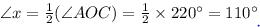∴ The measure of ∠x=110°

QUESTION: 9

In the figure below, PQ = QS, QR = RS and angle SRQ = 100°. How many degrees is angle QPS?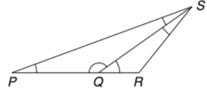Solution:

In DQRS; QR = RS, therefore ㄥRQS = ㄥRSQ (because angles opposite to equal sides are equal).
Thus, ㄥRQS + ㄥRSQ = 180° - 100° = 80°
ㄥRQS = ㄥRSQ = 40°
ㄥPQS = 180° – 40° = 140°
(sum of angles on a line = 180°)
Then again ㄥQPS = ㄥQSP (Since angles opposite to equal sides are equal)
Thus ㄥQPS + ㄥQSP = 180° – 140° = 40°
ㄥQPS = ㄥQSP = 20°

QUESTION: 10

In the given figure, AD is the bisector of –BAC, AB = 6 cm, AC = 5 cm and BD = 3 cm. Find DC. It is given that ∠ABD = ∠ACD.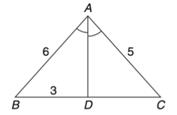Solution: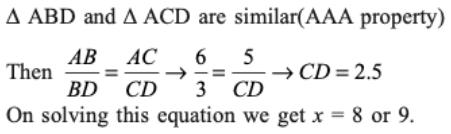QUESTION: 11

PQRS is a cyclic quadrilateral. If PQR is an equilateral triangle, find Angle ㄥRSP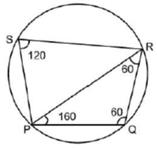Solution:

The sum of opposite angles of a cyclic quadrilateral is 180°. PQR is an equilateral triangle So, Measurement of all its angles is 60°.

ㄥPOR + ㄥRSP = 180°

60° + ㄥRSP = 180° ► ㄥRSP = 120°

QUESTION: 12

In a triangle ABC, the incentre is at 0. If ㄥBOC = 100°, find ㄥBAC.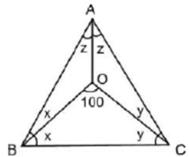Solution: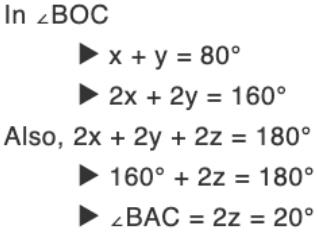QUESTION: 13

A rectangular enclosure 40 m x 36 m has a horse tethered to a corner with a rope of 14 m in length. What is the ratio of the respective areas it can graze, if it is outside the enclosure and if it is inside the enclosure?

Solution: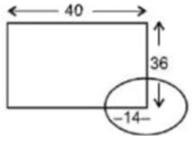Imagine a circle on the corner of the rectangle

3 quarters of the circle lie outside the rectangle and 1 quarter lies inside.

Hence Required ratio = 3 :1

QUESTION: 14

Read the passage below and solve the questions based on it.

The area of a square is equal to the area of a rectangle. Moreover, the perimeter of the square is also equal to the perimeter of the rectangle.

Q. The length of the rectangle is equal to the:

Solution:

This is possible only when both length and breadth of rectangle are equal to the side of the square.

QUESTION: 15

A quadrilateral is inscribed in a circle. If an angle is inscribed in each of the four segments outside the quadrilateral, then what is the sum of these four angles?

Solution: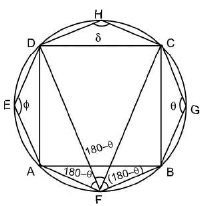Required sum
= θ + 180° - θ + 180° - θ + 180° - θ + θ + 8 = 540°